## You need to test, we're here to help.

You need to test, we're here to help.

## 28 February 2022

### Signal and Power Integrity Tutorial: A Robust Method for Measuring Clock Jitter with Oscilloscopes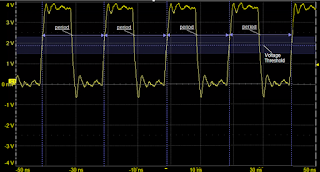Figure 1. Clock jitter measured as a variationof clock signal absolute period.
Clock jitter is the variation of a clock signal’s frequency or period. Either measurement carries the same information, but the period measurement is a simple time interval measurement easily performed using a real-time oscilloscope. If we have a robust way of measuring clock jitter, we have the basis for measuring the clock signal’s sensitivity to other features in the environment that can affect the period. Voltage noise on the power rail is just one external force that can affect clock jitter, which we'll show you how to measure in a future post.

In this post, we’ll demonstrate a robust method for measuring clock jitter using an example from Dr. Eric Bogatin’s webinar, “The Impact of Power Rail Noise on Clock Jitter.”

The clock in our examples is a 5-stage ring oscillator which generates a square wave signal between 10 and 66 MHz. The test instrument is a WavePro HD 12-bit, 4-Ch, 8 GHz, 20 GS/s, 5 Gpts oscilloscope with 60 fs sample clock jitter.

In the process, we make a series of oscilloscope sample clock tests and timebase adjustments as  consistency checks. While measuring jitter is less about absolute accuracy than about the relative precision of measuring the time interval from cycle to cycle, a fundamental part of that is ensuring the absolute accuracy of the oscilloscope’s timebase.

### 1. Test Oscilloscope Sample Clock Jitter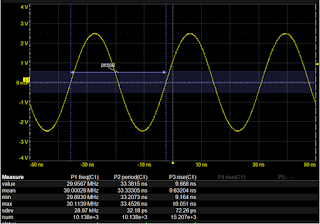Figure 2. Testing oscilloscope sample clock accuracy using a known signal source.
Before measuring the clock signal jitter, it’s advisable to do a “situational awareness” test of the oscilloscope’s timebase accuracy using a known source to verify it meets the specification. To do this, we use a synthesized function generator with 1 PPM absolute accuracy to generate a 30 MHz,
5 V signal and apply it to the oscilloscope on C1. We set a 50% Edge trigger and a fixed sample rate of 20 GS/s (Figure 2). After measuring the known source frequency, rise time and period using parameters, we calculate the fractional uncertainty in PPM using the formula: (Δf/f)*10^6. With a mean frequency of 30.00028 MHz, Δf =280 Hz, so the fractional uncertainty in PPM on a 30 MHz signal is 280/30*10^6 = 9.333, indicating the absolute accuracy of the oscilloscope timebase is under 10 PPM. Pretty good.

### 2. Estimate Expected Clock Period

Next, we switch the trigger to the clock source channel, and adjust the V/div and Time/div until two periods of the signal are visible on the graticule. Figure 3 shows the waveform of the ring oscillator on C2. The period is a little more than four divisions, which at 5 ns/division is about 21 ns. The rise time is a little less than two minor divisions, each of which represents 1 ns, so about 1.5 ns.

### 3. Measure Clock Period Using Parameters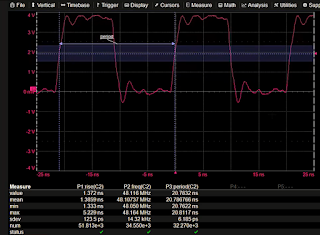Figure 3. Measuring clock signal rise time, frequency and period.
Using parameters with statistics on, we measure the rise time, frequency and period of the clock signal. The mean frequency is 48 MHz, corresponding to a period of 20.75 ns, very close to the estimated 21 ns, and within the specified 10 to 66 MHz. The estimated rise time was 1.5 ns, and the measured value is 1.38 ns.

### 4. Calculate the Standard Deviation of the Clock Period

The standard deviation (sdev) is a measure of the spread of values about the mean. By definition, in a Gaussian distribution, 68% of all measured values are within ±1 standard deviation from the mean. The period sdev value is a good figure of merit for clock jitter, which is a measure of variation from the mean.

With statistics on, the sdev of every parameter measurement is already calculated. Our clock period sdev measurement is 6.18 ps. However, since the oscilloscope’s intrinsic sample clock jitter is specified at 60 fs, and we have ascertained an accuracy of ~9 PPM, the measured period jitter at 6.18 ps is much higher than the fundamental limit of the oscilloscope. The measurement is likely “real.”

### 5. Increase the Timebase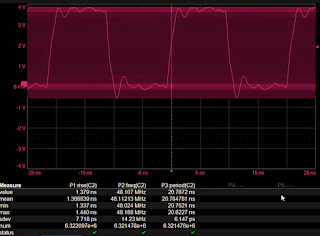Figure 4. Measuring clock rise time, frequency and period over a long acquisition. The parametersare measuring the full acquisition, not the zoomoverlaid on it.
Keeping the maximum fixed sample rate, we increase the Time/div to 20 µs/div for a total acquisition time of 200 µs and record length of 4M sample points. At 4M points per acquisition and over 6.3 million measurements in the buffer, our period measurement still has an sdev of 6.1 ps— around 6 ps every 20 ns period, or about 0.03% variation in the period. (Figure 4).

### 6. Track and Histogram the Period Measurement

Figure 5 shows both the track and the histogram of our period measurement. The track function is displayed over the acquired waveform, while the histogram is plotted in a separate grid.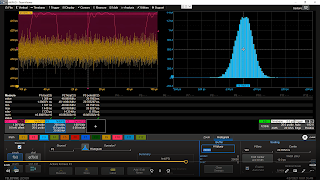Figure 5. Statistical analysis of period measurement using tracks and histograms.
The vertical scale of our track function is 10 ps/div, same as the horizontal scale of our histogram. Just by “eyeballing” the extent of the track, we can confirm the sdev matches the 6 ps sdev that is calculated by the period measurement statistics. The histogram is centered near the mean value of the period measurement at 20.7852 ns. The saddle shape of the histogram is characteristic of the Gaussian or Normal distribution, a good indication that the clock jitter is the result of a random process.

### 7. Lower Sample Rate Until Measurement Degrades

As a consistency check, we investigate how the oscilloscope’s sampling rate affects the measured clock jitter. This is done for “situational awareness,” to ensure that the instrumentation is not affecting the measurements. We lower the sample rate by successive steps until the measurement visibly degrades. From 20 GS/s, we step to 10 GS/s, 5 GS/s and 2.5 GS/s with little change in the measured period sdev or the shape of the histogram. Only when we reach 1 GS/s can we see a significant change (Figure 6).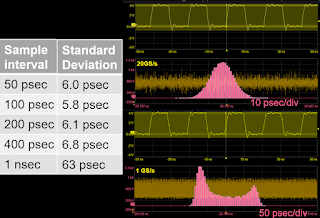Figure 6. Confirming the measurement over a range of sample rates.
At 1 GS/s, the edges of the clock signal are not well enough defined by the number of samples to measure the period accurately. There are only about 1.3 samples on the edge (the bright dots on the zoomed clock waveform in Figure 6). As a general rule, you should use the highest possible sampling rate for jitter measurements. However, this confirms that even at a fraction of our maximum sampling rate, measurement accuracy is good, and at 20 GS/s the jitter measurement is quite trustworthy.

Want to try this with your clock source? Download our step-by-step tutorial, A Robust Method for Measuring Clock Jitter.

You can also watch Dr. Eric Bogatin demonstrate in the on-demand webinar, “The Impact of Power Rail Noise on Clock Jitter.”